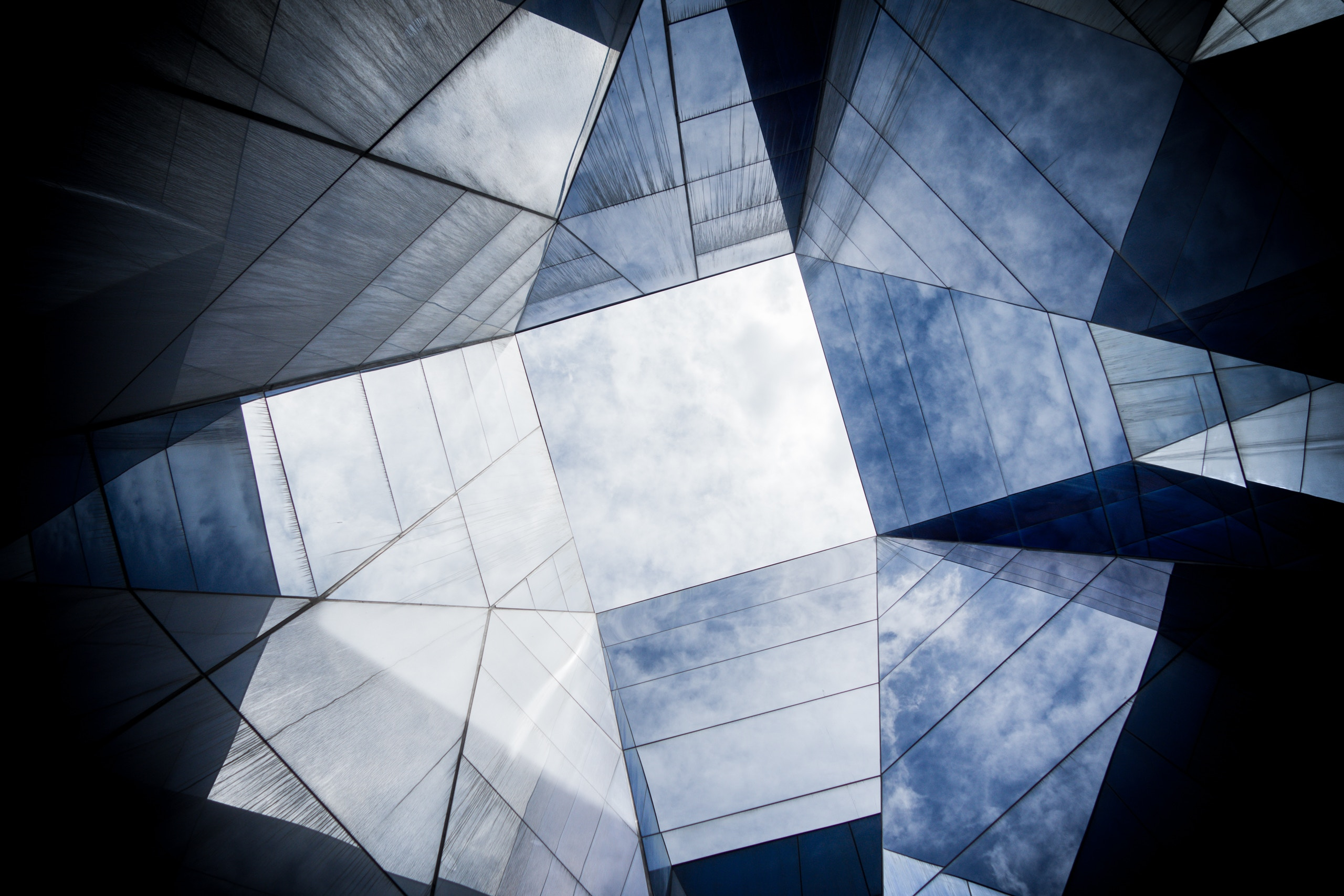# On degenerations of $\mathbb Z/2$-Godeaux surfaces

#### Preprint

We compute equations for Coughlan's family of Godeaux surfaces with torsion $\mathbb Z/2$, which we call $\mathbb Z/2$-Godeaux surfaces, and we show that it is (at most) 7 dimensional. We classify non-rational KSBA degenerations $W$ of $\mathbb Z/2$-Godeaux surfaces with one Wahl singularity, showing that $W$ is birational to particular either Enriques surfaces, or $D_{2,n}$ elliptic surfaces, with $n=3,4$ or $6$. We present examples for all possibilities in the first case, and for $n=3,4$ in the second.

### Publication

Year of publication: 2020

### Identifiers

Other: arXiv:2002.08836v1